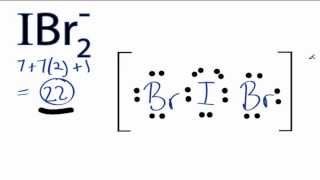# What are the bond angles in the central atom of the following: "NSF", "OF"_2, and "IBr"_2^−"?

Dec 17, 2015

The bond angles are slightly less than 120°, slightly less than 109.5 °, and 180 °, respectively.

#### Explanation:

$\boldsymbol{\text{NSF}}$

The structure of $\text{NSF}$ isThere is a lone pair on the $\text{N}$ atom, a lone pair on the $\text{S}$ atom, and there are three lone pairs on the $\text{F}$ atom.

This is an $\text{AX"_2"E}$ molecule, so the electron geometry is trigonal planar and the molecular shape is bent.

The theoretical bond angle is 120 °, but repulsion by the lone pairs decreases the bond angle to about 117 °.

${\boldsymbol{\text{OF}}}_{2}$

The Lewis structure of ${\text{OF}}_{2}$ isThis is an ${\text{AX"_2"E}}_{2}$ molecule, so the electron geometry is tetrahedral and the molecular shape is bent.

The theoretical bond angle is 109.5 °, but repulsions by the lone pairs decrease the bond angle to about 103 °.${\boldsymbol{\text{IBr}}}_{2}^{-}$

The Lewis structure of ${\text{IBr}}_{2}^{-}$ isThis is an ${\text{AX"_2"E}}_{3}$ structure, so the electron geometry is trigonal bipyramidal.The lone pairs occupy the equatorial positions, with the $\text{Br}$ atoms in the axial locations.

The bond angle is 180 °.